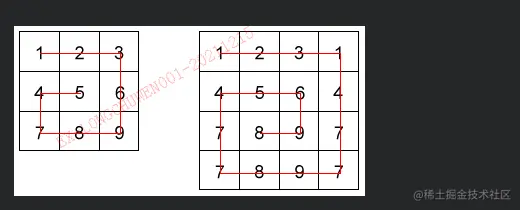# Python snail sorting

2022-02-02 07:24:18

Given a `n` x `n` Array , Returns the array elements arranged from the outermost element to the middle element , Move clockwise . for example ：

``````array = [[1,2,3],
[4,5,6],
[7,8,9]]
snail(array) #=> [1,2,3,6,9,8,7,4,5]
Copy code ``````
``````array = [[1,2,3],
[8,9,4],
[7,6,5]]
snail(array) #=> [1,2,3,4,5,6,7,8,9]
Copy code ``````

The specific sorting is shown in the figure ：## Python Code implementation

``````#  Snail sorting
array = [
[1, 2, 3],
[4, 5, 6],
[4, 5, 6]
]

def snail(arrays):
arr_list = cycle(arrays)
arr = arr_list
lists = arr_list

while len(arr) != 0:
#  Perform snail sorting
arr_list = cycle(arrays)
arr = arr_list
lists += arr_list
# print(lists)
return lists

def cycle(arrays):
arr_list = arrays
#  Get list length
length = len(arr_list)
lists = []
popList = []
for i in range(length):
#  The first list is sorted normally
if i == 0:
[lists.append(i) for i in arr_list[i]]
#  Get the last digit of the list except the first one
elif i < length:
lists.append(arr_list[i][length - 1])
arr_list[i].pop(length - 1)
#  Remove sorted numbers
popList.append(arr_list[i].pop(0))
#  Reverse the last list
if i == length - 1:
arr_list[i].reverse()
[lists.append(i) for i in arr_list[i]]

#  Invert the throw list and fill in the sort
popList.reverse()
for i in popList:
lists.append(i)

#  Make the function jump out of the loop
arr_list.pop(0)
length = len(arr_list)
if length == 0:
pass
else:
arr_list.pop(length - 1)

return lists, arr_list

print(snail(array))
Copy code ``````